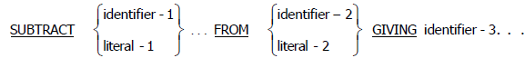## Thursday, 14 August 2014

### COBOL Subtract Statement | SUBTRACT STATEMENT Example.

Numerical calculation is one of the most critical part of programming language. In-fact most of the programming language provide various functions that can be used to implement business calculation logic.

Similarly, COBOL also provide various clause such as SUBTRACT, MULTIPLY, ADD, DIVIDE, COMPUTE etc to perform various calculation

In this tutorial, I would focus on various format of SUBTRACT clause available in COBOL. I would try to explain syntax with few examples.

The SUBTRACT statement subtracts one numeric item, or the sum of two or more numeric items, from one or more numeric items and stores the result. Subtract has three different format which is applicable in various situation.

Format : 1COBOL Subtract From.
Example:
```
```
`SUBTRACT 1 FROM WS-COUNTER.`
```
```
Here, all identifiers or literals preceding the key word FROM are added together and this sum is subtracted from and stored immediately in identifier-2. This process is repeated for each successive occurrence of identifier-2, in the left -to-right order in which identifier-2 is specified.
Format : 2COBOL Subtract From Giving
Example:
`SUBTRACT WS-HOURS FROM TOT-HOURS GIVING OVERTIME-HRS. `
Here, all identifiers or literals preceding the key word FROM are added together and this sum is subtracted from identifier-2 or literal-2. The result of the subtraction is stored as the new value of each data item referenced by identifier-3.
Format : 3Subtract From in COBOL
Example:

`SUBTRACT WS-AMT1 WS-AMT2 WS-AMT3 FROM GRS-PAY GIVING NET-PAY.`
Here, the elementary data items within identifier-1 are subtracted and stored in the corresponding elementary items within identifier-2.

#### Check-out my tutorial on COBOL BLANK WHEN ZERO Clause.